Question

# Calculating the pH at equivalence of a titration A chemist titrates 120.0 mL of a 0.7513...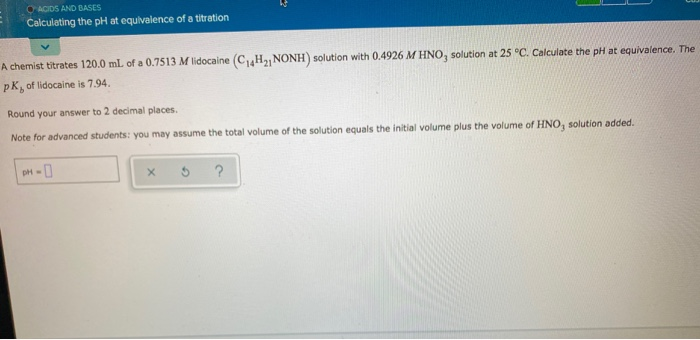Calculating the pH at equivalence of a titration A chemist titrates 120.0 mL of a 0.7513 M lidocaine (C4H2, NONH) solution with 0.4926 M HNO, solution at 25°C. Calculate the pH at equivalence. The PK, of lidocaine is 7.94 Round your answer to 2 decimal places Note for advanced students: you may assume the total volume of the solution equals the initial volume plus the volume of HNO, solution added. DH-

base = 120 x 0.7513 = 90.156

volume of salt at equivalence point   = 90.156 / 0.4926 = 183. 0 m
salt concentration = 90.156 / 183 +120 = 0.2975 M

pH = 7 - 1/ 2[pKb + log C]

= 7 - 1/2 [7.94 + log 0.2975 ]

= 3.29

pH = 3.29

#### Earn Coins

Coins can be redeemed for fabulous gifts.

Similar Homework Help Questions
• ### - Calculating the pH at equivalence of a titration A chemist titrates 180.0 ml of a...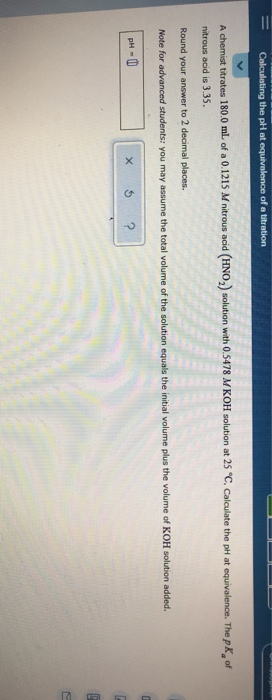- Calculating the pH at equivalence of a titration A chemist titrates 180.0 ml of a 0.1215 M nitrous acid (HNO) solution with 0.5478 M KOH solution at 25 "C. Calculate the pH at equivalence. The pk of nitrous acid is 3.35. Round your answer to 2 decimal places. Note for advanced students: you may assume the total volume of the solution equals the initial volume plus the volume of KOH solution added. PHU X 5 ?

• ### ACIDS AND BASES Calculating the pH at equivalence of a titration A chemist titrates 130.0 mL...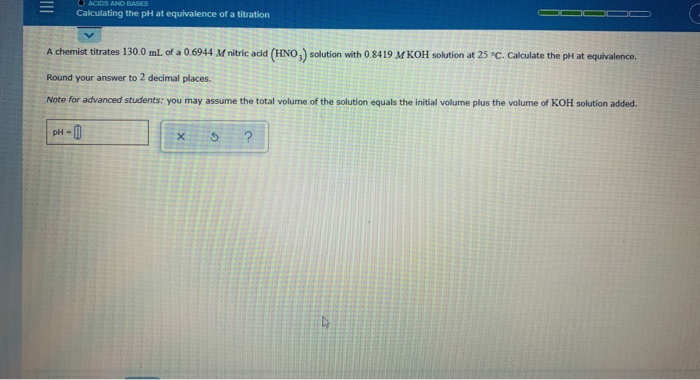ACIDS AND BASES Calculating the pH at equivalence of a titration A chemist titrates 130.0 mL of a 0.6944 M nitric acid (HNO.) solution with 0.8419 M KOH solution at 25 °C. Calculate the pH at equivalence Round your answer to 2 decimal places. Note for advanced students: you may assume the total volume of the solution equals the initial volume plus the volume of KOH solution added. pH-0 X 5 ?

• ### = O ACIDS AND BASES Calculating the pH at equivalence of a titration A chemist titrates...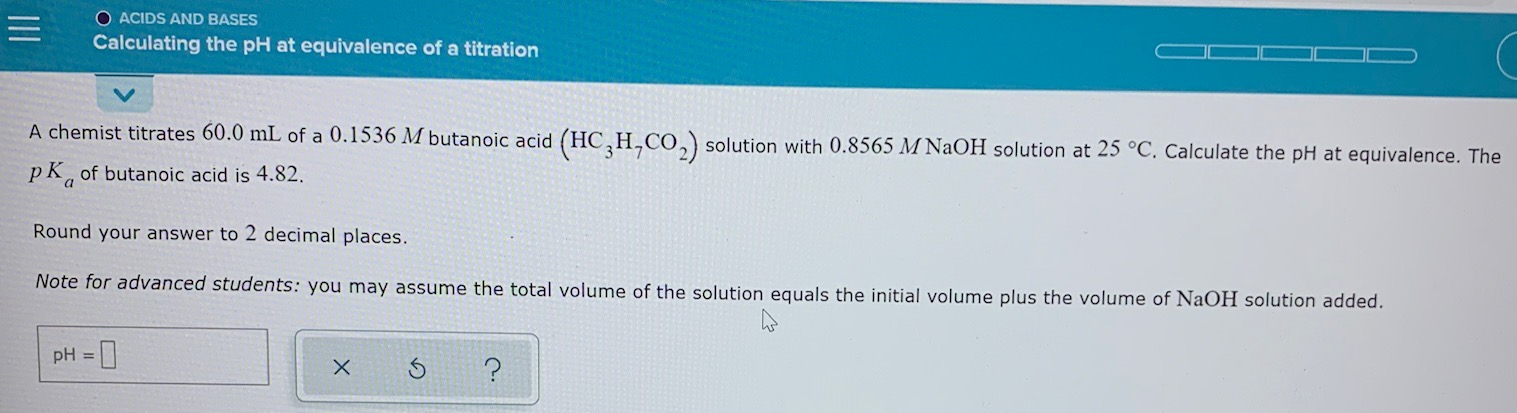= O ACIDS AND BASES Calculating the pH at equivalence of a titration A chemist titrates 60.0 mL of a 0.1536 M butanoic acid (HC,H,CO2) solution with 0.8565 M NaOH solution at 25 °C. Calculate the pH at equivalence. The pk, of butanoic acid is 4.82. Round your answer to 2 decimal places. Note for advanced students: you may assume the total volume of the solution equals the initial volume plus the volume of NaOH solution added. pH = x...

• ### ACIDS AND BASES Calculating the pH at equivalence of a titration A chemist titrates 200.0 mL...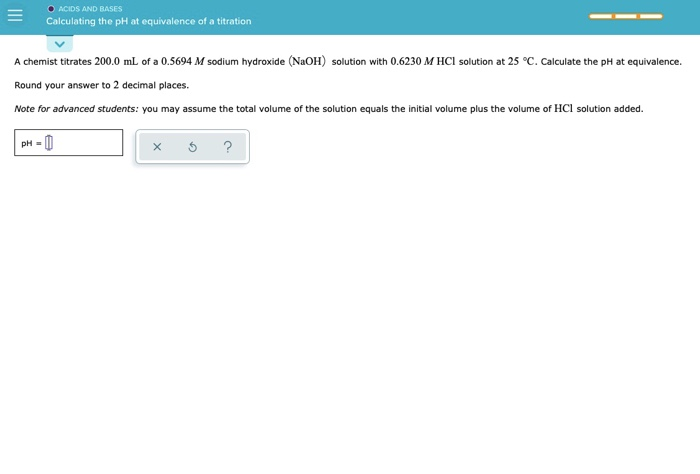ACIDS AND BASES Calculating the pH at equivalence of a titration A chemist titrates 200.0 mL of a 0.5694 M sodium hydroxide (NaOH) solution with 0.6230 M HCl solution at 25 °C. Calculate the pH at equivalence. Round your answer to 2 decimal places. Note for advanced students: you may assume the total volume of the solution equals the initial volume plus the volume of HCl solution added. PH-0 5 ?

• ### A chemist titrates 250.0 mL of a 0.5659 M lidocaine (C14H21NONH) solution with 0.4276 M HBr...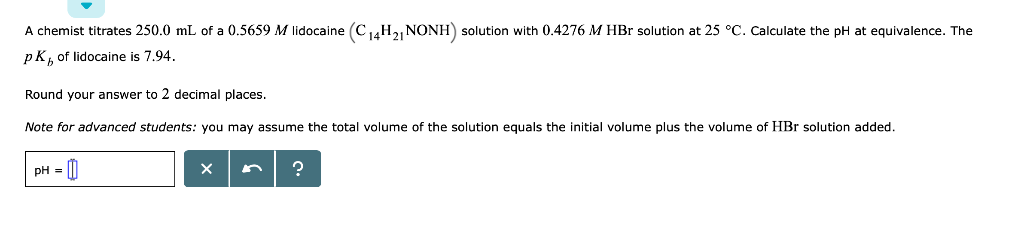A chemist titrates 250.0 mL of a 0.5659 M lidocaine (C14H21NONH) solution with 0.4276 M HBr solution at 25 °C. Calculate the pH at equivalence. The pK, of lidocaine is 7.94. Round your answer to 2 decimal places Note for advanced students: you may assume the total volume of the solution equals the initial volume plus the volume of HBr solution added. PH -

• ### Calculating the pH at equi.. A chemist titrates 70.0 ml. of a 04557 M carbonic acid...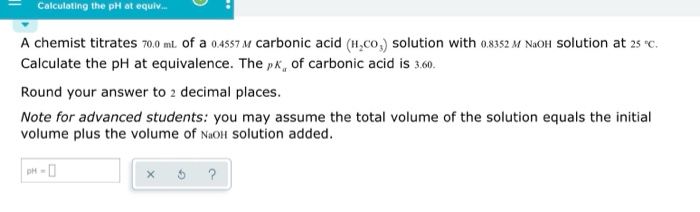Calculating the pH at equi.. A chemist titrates 70.0 ml. of a 04557 M carbonic acid (H,co,) solution with o8352 M NaOlH Solution at 2s c Calculate the pH at equivalence. The pk, of carbonic acid is 3.60 Round your answer to 2 decimal places. Note for advanced students: you may assume the total volume of the solution equals the initial volume plus the volume of NaoH Solution added. рH - D

• ### A chemist titrates 50.0 ml. of a 0.6210 Maniline (CH NH) solution with 0.2970 M HNO,...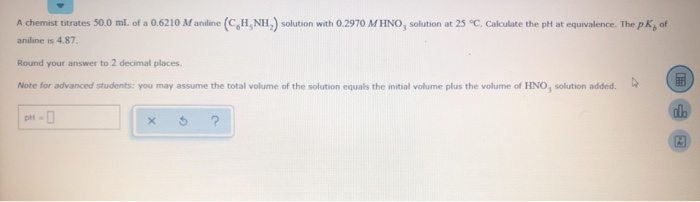A chemist titrates 50.0 ml. of a 0.6210 Maniline (CH NH) solution with 0.2970 M HNO, solution at 25°C. Calculate the ph at equivalence. The pK, of aniline is 4.87 Round your answer to 2 decimal places. Note for advanced students: you may assume the total volume of the solution equals the initial volume plus the volume of HNO, solution added. pH- x 5 ?

• ### A chemist titrates 90.0 mL of a 0.5705 M carbonic acid (H,CO,) solution with 0.6274 M...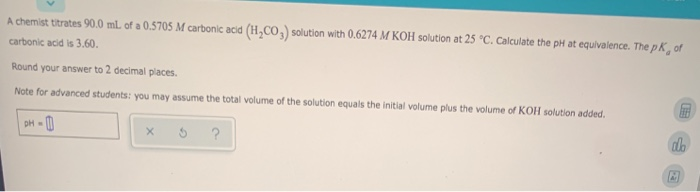A chemist titrates 90.0 mL of a 0.5705 M carbonic acid (H,CO,) solution with 0.6274 M KOH solution at 25 °C. Calculate the pH at equivalence. The pk of carbonic acid is 3.60. Round your answer to 2 decimal places. Note for advanced students: you may assume the total volume of the solution equals the initial volume plus the volume of KOH solution added. DH-

• ### A chemist titrates 90.0mL of a 0.3683M dimethylamine CH32NH solution with 0.8804M HNO3solution at 25°C. Calculate...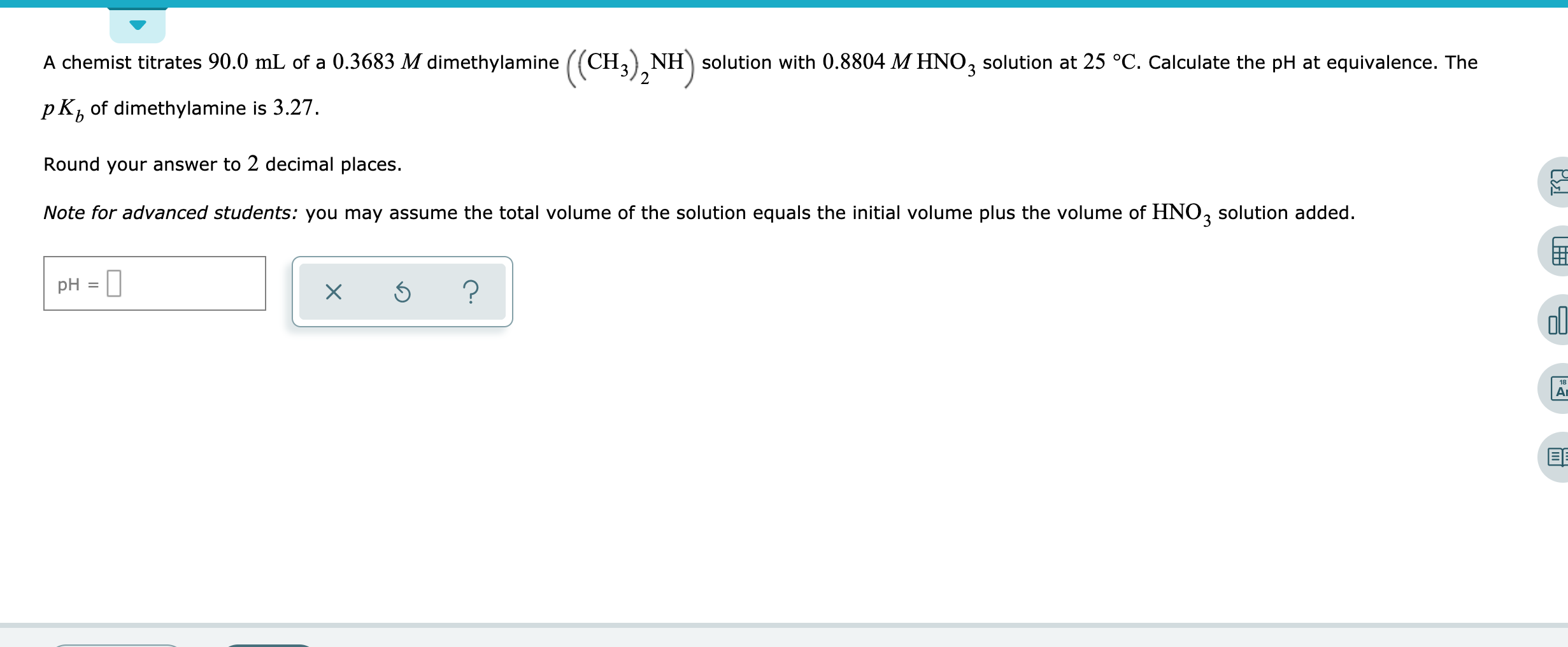A chemist titrates 90.0mL of a 0.3683M dimethylamine CH32NH solution with 0.8804M HNO3solution at 25°C. Calculate the pH at equivalence. The pKb of dimethylamine is 3.27. Round your answer to 2 decimal places. A chemist titrates 90.0 mL of a 0.3683 M dimethylamine 1 solution with 0.8804 M HNO, solution at 25 °C. Calculate the pH at equivalence. The pK, of dimethylamine is 3.27. Round your answer to 2 decimal places. Note for advanced students: you may assume the total...

• ### A chemist titrates 150.0 mL of a 0.4938 M sodium hydroxide (NaOH) solution with 0.6911 M...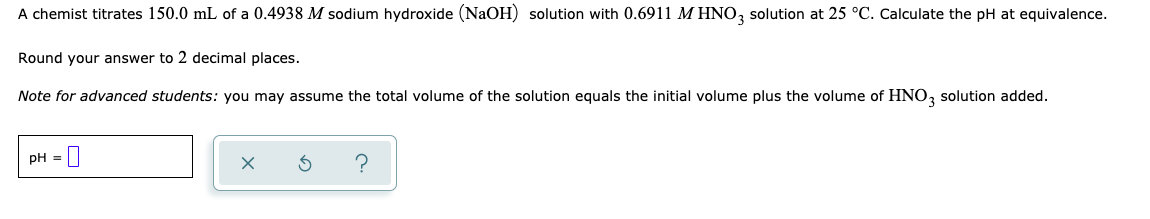A chemist titrates 150.0 mL of a 0.4938 M sodium hydroxide (NaOH) solution with 0.6911 M HNO, solution at 25 °C. Calculate the pH at equivalence. Round your answer to 2 decimal places. Note for advanced students: you may assume the total volume of the solution equals the initial volume plus the volume of HNO2 solution added. pH = 0